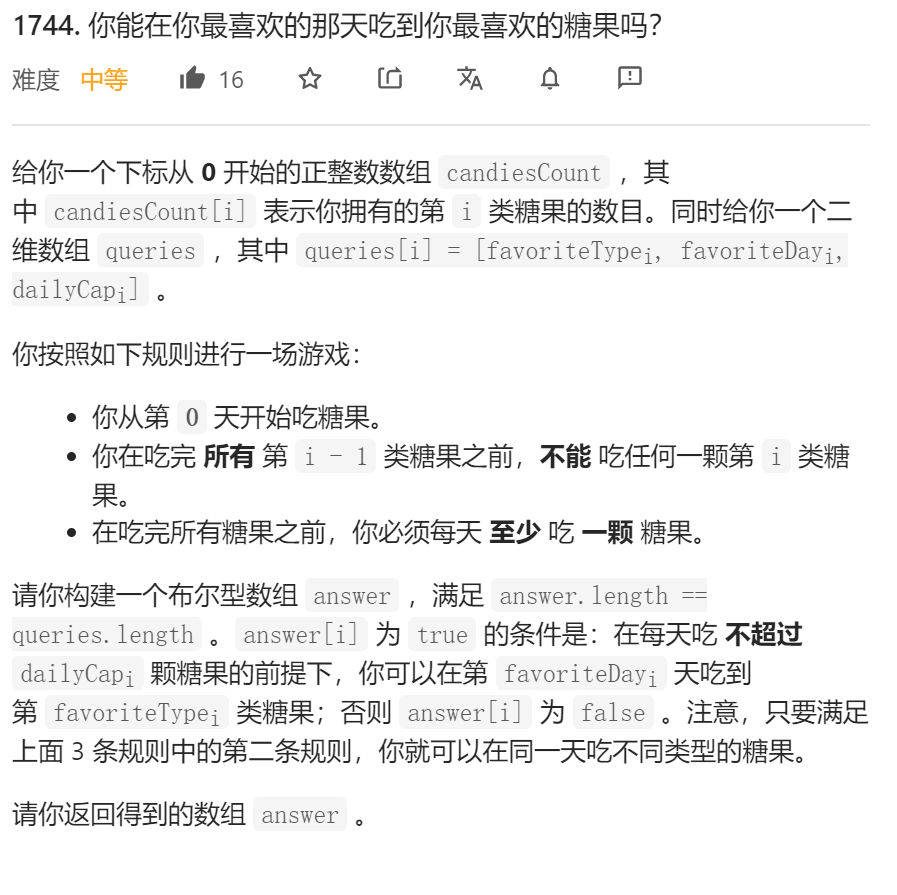﻿• 价格透明
• 信息保密
• 进度掌控
• 售后无忧• 题目
• 题目的解读
• 解题代码

# 题目# 题目的解读

1. 你从第 0 天开始吃糖果。
2. 你在吃完 所有 第 i - 1 类糖果之前，不能 吃任何一颗第 i 类糖果。
3. 在吃完所有糖果之前，你必须每天 至少 吃 一颗 糖果。

`至此我们只需要计算这两个区间是否含有交集，一旦含有交集，就能在最喜欢的那天吃到最喜欢的糖果`

# 解题代码

``````class Solution {
public:
vector<bool> canEat(vector<int>& candiesCount, vector<vector<int>>& queries) {
int n = candiesCount.size();
long long sum[n];
memset(sum,0,sizeof(sum));
//让sum[i]表示类型0一直到类型i的糖果的总数(需要long long防止溢出)
for(int i=0;i<n;i++){
sum[i] = candiesCount[i];
if(i>0)
sum[i] += sum[i-1];
}
vector<bool>res;
for(auto q:queries){
int favType = q;
int favDay = q;
int dayCap = q;
//开始计算需要的范围
long long x1 = favDay+1;
long long y1 = (long long)(favDay+1)*dayCap;
long long x2 = favType==0?1:sum[favType-1]+1;
long long y2 = sum[favType];
//很明显，当y1<x2或者是y2<x1时无任何交集
res.push_back(!(y1<x2||y2<x1));
}
return res;
}
};

``````### 低价透明### 金牌服务### 信息保密